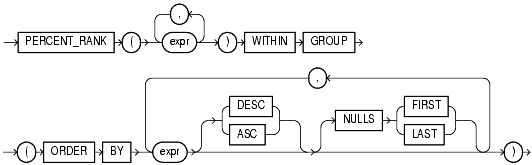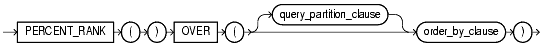## PERCENT_RANK

#### Aggregate Syntax

percent_rank_aggregate::=Text description of percent_rank_aggregate

#### Analytic Syntax

percent_rank_analytic::=Text description of percent_rank_analytic

#### Purpose

`PERCENT_RANK` is similar to the `CUME_DIST` (cumulative distribution) function. The range of values returned by `PERCENT_RANK` is 0 to 1, inclusive. The first row in any set has a `PERCENT_RANK` of 0.

• As an aggregate function, `PERCENT_RANK` calculates, for a hypothetical row R identified by the arguments of the function and a corresponding sort specification, the rank of row R minus 1 divided by the number of rows in the aggregate group. This calculation is made as if the hypothetical row R were inserted into the group of rows over which Oracle is to aggregate. The arguments of the function identify a single hypothetical row within each aggregate group. Therefore, they must all evaluate to constant expressions within each aggregate group. The constant argument expressions and the expressions in the `ORDER` `BY` clause of the aggregate match by position. Therefore the number of arguments must be the same and their types must be compatible.
• As an analytic function, for a row R, `PERCENT_RANK` calculates the rank of R minus 1, divided by 1 less than the number of rows being evaluated (the entire query result set or a partition).

#### Aggregate Example

The following example calculates the percent rank of a hypothetical employee in the sample table `hr.employees` with a salary of \$15,500 and a commission of 5%:

```SELECT PERCENT_RANK(15000, .05) WITHIN GROUP
(ORDER BY salary, commission_pct) "Percent-Rank"
FROM employees;

Percent-Rank
------------
.971962617
```

#### Analytic Example

```The following example calculates, for each employee, the percent rank of the
employee's salary within the department: SELECT department_id, last_name,
salary,
PERCENT_RANK()
OVER (PARTITION BY department_id ORDER BY salary DESC) AS pr
FROM employees
ORDER BY pr, salary;

DEPARTMENT_ID LAST_NAME                     SALARY         PR
------------- ------------------------- ---------- ----------
10 Whalen                          4400          0
40 Marvis                          6500          0
.
.
.
80 Vishney                        10500 .176470588
50 Everett                         3900 .181818182
30 Khoo                            3100         .2
.
.
.
80 Johnson                         6200 .941176471
50 Markle                          2200 .954545455
50 Philtanker                      2200 .954545455
50 Olson                           2100          1
.
.
.
```